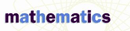# Scientific Notation - Research Article from Macmillan Science Library: Mathematics

This encyclopedia article consists of approximately 3 pages of information about Scientific Notation.
 This section contains 586 words (approx. 2 pages at 300 words per page) View a FREE sampleScientific notation is a method of writing very large and very small numbers. Ordinary numbers are useful for everyday measurement, such as daily temperatures and automobile speeds, but for large measurements like astronomical distances, scientific notation provides a way to express these numbers in a short and concise way.

The basis of scientific notation is the power of ten. Since many large and small numbers have a few integers with many zeros, the power of ten can be used to shorten the length of the written number.

A number written in scientific notation has two parts. The first part is a number between 1 and 10, and the second part is a power of ten. Mathematically, writing a number in scientific notation is the expression of the number in the form n × 10x where n is a number greater than 1 but less than 10 and x is an exponent...

(read more)

 This section contains 586 words (approx. 2 pages at 300 words per page) View a FREE sampleMore summaries and resources for teaching or studying Scientific Notation.
CopyrightsScientific Notation from Macmillan. Copyright © 2001-2006 by Macmillan Reference USA, an imprint of the Gale Group. All rights reserved.